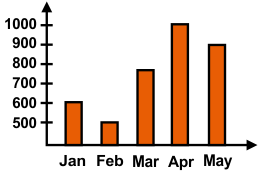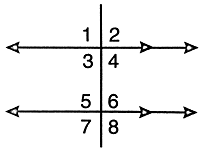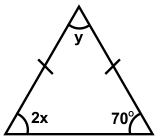﻿ Maths Olympiad (CMO) Previous Year Paper for Class 6

# CREST Mathematics Olympiad Class 6 Previous Year Papers

CREST Mathematics Olympiad Previous Year Paper PDF for Class 6:

## Syllabus:

Section 1: Knowing Our Numbers, Whole Numbers, Playing with Numbers, Basic Geometrical Ideas, Understanding Elementary Shapes, Integers, Fractions, Decimals, Data Handling, Mensuration, Algebra, Ratio And Proportion, Symmetry, Practical Geometry.

Achievers Section: Higher Order Thinking Questions - Syllabus as per Section 1

 Q.1 What is the product of 8/11 and the additive inverse of 44/2?
 Q.2 It takes 90 minutes to wash 20 vehicles at a car wash. At this rate, how many minutes does it take to wash 5 vehicles?
 Q.3 The bar graph shows the sale of chocolates in 5 months from January to May: What is the total sale of chocolates from January to May?Q.4 How many lines of symmetry are there in an equilateral triangle?
 Q.5 George travels from place A to place B and Peter travels from place B to place A. George travels one-third of the distance and Peter travels one-fourth of the distance. The distance travelled by George is one kilometre more than the distance travelled by Peter. What is the distance between place A and place B? (In km)
 Q.6 The average marks in Mathematics for 5 students were found to be 50. Later it was discovered that in the case of one student, the marks 48 were misread as 84. The correct average is:
 Q.7 In the given figure ∠1 = x and ∠7 = 2x/3. Find the value of ∠5:Q.8 Fill in the blank: The number of diagonals in a heptagon is _________.
 Q.9 Consider the following quotients: I. 368.39 divided by 17 II. 170.50 divided by 62III. 875.65 divided by 83Their correct sequence in decreasing order is:
 Q.10 Consider an isosceles triangle as shown in the figure below. Find the value of x + y:Q.1 a Q.2 c Q.3 b Q.4 d Q.5 c Q.6 c Q.7 c Q.8 d Q.9 a Q.10 c

Students can download the CREST Mathematics Olympiad previous year paper pdf for class 6 from this page. Each question in the paper carries 1 mark. The answer key for all the questions is also provided on the last page.

Students must become familiar with the exam format before taking any competitive exam, such as an Olympiad. CREST Mathematics Olympiad previous year paper will help students to become familiar with the exam pattern.

Once students complete their syllabus, they must start solving the CREST Mathematics Olympiad previous year paper for class 6 to analyze their preparation level. This is the proven way to excel in the CREST Mathematics Olympiad examination.